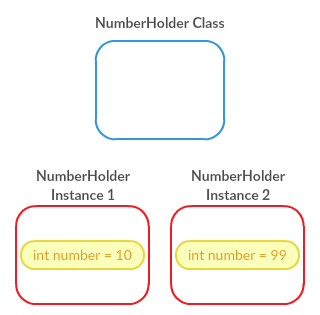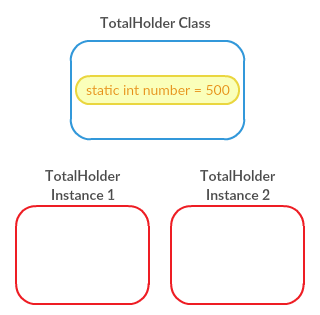JBay Solutions Development Blog on Java, Android, Play2 and others

A very common question from beginners in Java is "What does Static means" or "What is Static in Java". It is actually very simple.

Something that is Static in Java is something that belongs to the Class and not the objects of that Class type, hence it is shared across the instances of that Class type.

To better understand what is meant by that, let us rewind a bit!

When writing a Java program, a programmer writes Classes and creates objects of those Class types, or other Class types that belong to some libs. So, look at this Class:

``````public class NumberHolder {

public int number = 5;

}
``````

This Class simply holds a number in a public variable called `number`. If we wanted to use this class we would need to instantiate this class to create an object, and then use that object to store the value on the variable, just like so :

``````NumberHolder holder1 = new NumberHolder();
holder1.number = 10;
``````

If we would print the value of the "number" variable inside the holder1 object like so:

``````System.out.println("Value of holder1 number : " + holder1.number);
``````

we would get:

``````Value of holder1 number : 10
``````

Now, if we wanted to hold two numbers, we would need to create two objects of type `NumberHolder` and assign the values to them, like this:

``````NumberHolder holder1 = new NumberHolder();
holder1.number = 10;

NumberHolder holder2 = new NumberHolder();
holder2.number = 99;
``````

Printing the values of the number variables inside of each of the `NumberHolder` objects :

``````System.out.println("Value of holder1 number : " + holder1.number);
System.out.println("Value of holder2 number : " + holder2.number);
``````

Would produce :

``````Value of holder1 number : 10
Value of holder2 number : 99
``````

So, each of the objects has a variable that belongs to that specific object of class type `NumberHolder`.

Now in some cases there is a need to have a value that is shared across every instance of a particular class, and to do this, we use Static. Now, bear in mind that making something Static has several implications, that we'll see a bit further down.

## Something that is static

So, now we'll create another class that we'll call `TotalHolder` , which looks somewhat like this:

``````public class TotalHolder {

public static int total = 0;

}
``````

Very simple. Now, we'll create two instances of `TotalHolder` and do some assigning to the total int variable and see what happens:

``````TotalHolder totalHolder1 = new TotalHolder();
TotalHolder totalHolder2 = new TotalHolder();

totalHolder1.total = 10;
System.out.println("Print total through totalHolder1 : " + totalHolder1.total);

totalHolder2.total = 500;
System.out.println("Print total through totalHolder2 : " + totalHolder2.total);

System.out.println("Print total again through totalHolder1 : " + totalHolder1.total);
``````

If we run this code we get an output like this:

``````Print total through totalHolder1 : 10
Print total through totalHolder2 : 500
Print total again through totalHolder1 : 500
``````

So, as you can see, the value of the variable `total`, when being accessed through either of the `TotalHolder` instances will have the same value..... that is because it is exactly the same variable that is being accessed, not two different variables that share the same value, but the same exact variable! In fact, the static variables (or methods) don't even belong to the Instances of objects, but to the Class itself... and to illustrate that I've made two pictures :This last image show the example using the NumberHolder objects. As you can see, each instance of the NumberHolder has its own independent "number" variable.

As for the Static example, look at this image:Because the variable `total` is static, it does not belong to the Instances of the `TotalHolder`, but to the class itself. Which means that when accessed, through whatever instance of the object, it will be in fact the Class variable that is being accessed.

But that is not all..... because the static variables (and methods) belong to the Class, we don't really need to have Instances of the objects to access those variables, which means that the previous example code, code be re-written like this:

``````TotalHolder.total = 10;
System.out.println("Print total through TotalHolder Class : " + TotalHolder.total);

TotalHolder.total = 500;
System.out.println("Print total through     TotalHolder Class : " + TotalHolder.total);

System.out.println("Print total again through TotalHolder Class : " + TotalHolder.total);
``````

Notice that in this example we are not using any Instances, but instead accessing the `total` variable through the Class... which is completely legal, because the variable belongs to the class.... because it is static.... is it making sense?

But wait !!!!!!! This is not all !!!!! What about methods ?

Methods can also be static, and the same story applies to those methods! Methods that are static belong to the Class, and methods that are not static belong to the instance.... Things might be starting to sound strange, but don't give up on this! It is actually quite simple and I'll try to explain it with examples now.

## Static Methods? Why ?

OK, lets recap a bit, cause this really needs to be second nature:

• Static variables belong to the Class
• Static methods belong to the Class
• Static methods and variables can be accessed directly through the Class, instead of Instances.
• Non-static methods and variables belong to their Instances, and can only be accessed through those instances

So, to have have a variable that is shared with all Instances of a Class type, we can use a static Variable.

But what about methods? What are the benefits of having Static methods? Are those really needed?

We'll focus on the benefits first!

Because a Static method can be accessed through the Class, there is no need to create an Instance of the class to get access to the methods, which can be very useful with memory management... less instances, less memory used, less GC , less everything! But this is not all. In terms of code style and code reading.... it just makes everything much more readable and easy to understand, if used properly.

One good, and very common use of Static methods, is to create Util classes. Util classes is not an official designation I think, but what I mean by that is a Class that has useful methods (that are usually isolated) to perform certain common tasks, such as for example a Math util class, which could look like this:

``````public class MathUtil {

public static double multiply( double par1, double par2) {
return par1*par2 ;
}

public static double divide( double par1, double par2) {
return par1/par2 ;
}

public static double add( double par1, double par2) {
return par1-par2 ;
}

public static double subtract( double par1, double par2) {
return par1-par2 ;
}
}
``````

This is obviously a very simple class, but you get the idea! To use this class to make some operations, we would do something like this:

``````MathUtil.multiply( 20 , 2);
MathUtil.divide( 20 , 2);
MathUtil.subtract( 2 , 1);
``````

Really easy to read, isn't it? And notice, because we didn't create an Instance of the `MathUtil` Class, we are actually saving memory! Now imagine that in the ultra massive bank accounting system that you are developing using Java, if these methods were not static, and these operations needed to be done in several places, all the time, you would be creating `MathUtil` Instances all over the place, you would eventually use a considerable amount of memory, which would also at some point would need to be Garbage Collected..... and not to speak of the object Instantiation times.... you see? Static methods are a solution to these issues. Obviously that in a concurrent environment you would also need to have in mind concurrency considerations, but that is beyond the scope of this tutorial ( Check this Concurrency Tutorial if you are interested ) .

The big rule with Static methods is that, if you are accessing anything outside this method, that something also needs to be Static.

And this makes sence.... lets think about this for a second: If you are calling a Static method, and you know that a static method belongs to a Class and it can be accessed directly through the Class, if that method were to access a non-static variable, which would belong to a specific Instance, the VM wouldn't know which of the instance variables it was refering to, or even worst, if there were no Instances at all created, what would happen?

So, as you can see, having a static method accessing variables or methods outside of the scope of the method is illegal and your code won't compile... because it makes no sense.

And yes... there is more! Lets talk about static blocks!

## Static Blocks

You know what a block is, and static block is not very different and looks like this:

``````public class TotalHolder {

static {
total = 0;
}

public static int total;

}
``````

Think of static blocks that a Class can have as a Constructor for the Class. What happens is this: When you start you program on the JVM, classes get loaded into memory, this is where the Classes will live, and will be available to create instances, and access static variables and methods.

When a Class is loaded into memory, the JVM will initialize the static variables that exist on the Class, and will execute all the static blocks of the Class, in the order they are presented, always in that order.

Obviously, static block behave like static methods in the sense that they can only access static variables and methods that exist outside of the scope of the block, because.... specially at this time, when the static blocks are being executed, there will be no instances of those classes.

And this is it! We hope this was useful, and leave us any comment you would like to share or any doubts you may still have.